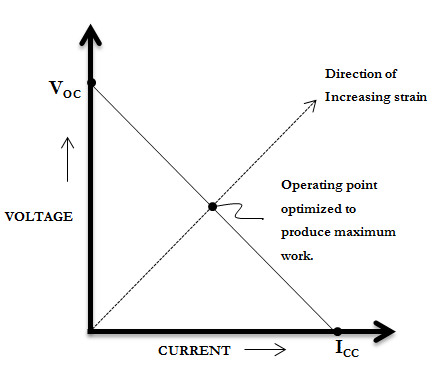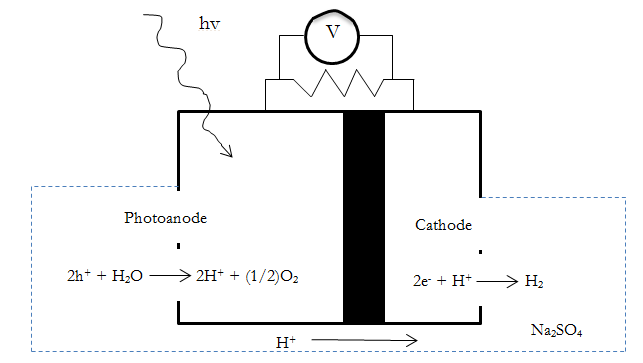# USE OF PIEZOELECTRIC GENERATOR (PEG) AND SOLAR POWER IN HOUSEHOLD APPLICATIONS – Memon Kashif.S & Kakde Anuja.B

Title: USE OF PIEZOELECTRIC GENERATOR (PEG) AND SOLAR POWER IN HOUSEHOLD APPLICATIONS

Authors: Memon Kashif.S, 3rd year, B.E,  Mechanical Engineering

Kakde Anuja.B, 3rd year, B.E, Information and Technology Engineering

College: Sinhgad College of Engineering, Pune

1. ABSTRACT
Depleting conventional resources and increasing demand of energy has directed to innovative methods to harness the non-conventional sources of energy. This paper aims at utilizing the power developed by solar energy and piezoelectric generators, together, in household appliances. The method involves use of Piezo Film on the floors of house and Solar Panels on roof, together charging the batteries to store energy and then utilizing this electric power for some household appliances. The design considerations and other governing parameters are discussed in this paper. This idea can be implemented in real life, reducing the stress on conventional resources. The purpose of this paper is to reduce the use of conventional electric power and an attempt to make a self-sustainable house.
2. KEYWORDS : Piezofilm, PZT, Piezoelectric generators, self-sustainable house, photoelectrochemical cell, Energy harvesting.

3. INTRODUCTION
With increasing work in automation and developments in E&TC sector, more and more electric power is demanded. But, the conventional sources like fuel are depleting day by day. Therefore, any alternative which uses non-conventional energy resources, is being tested. The need, to use piezoelectric effect and research on improving their performance, is being felt nowadays. The use of piezo to generate mass electricity is restricted due to their high impedance and low power output. To improve the power output, many piezo films are stacked together and the output voltage level is increased. This energy can be stored in batteries.
Also, the output power due to solar energy harvesting, can also be stored in photoelectrochemical cells. These cells use solar energy to perform electrolysis of water, and separate H2 and O2 , which can be stored. These gases are then recombined to produce electricity in a fuel-cell. Also, to increase the power generation due to solar energy, a steam turbine can be installed within a locality.

4. CONCEPT
The concept utilized in this paper is discussed below. Stacks of piezo films are used on the house floor, if not the entire house, then, at least in the region where chances of pressurizing the stacks are high e.g. in kitchen, at the doorsteps, staircases, living room, below chairs and beds, etc. The batteries kept in the vicinity of these strips can be charged. All these batteries along with the power generated by photoelectrochemical cell or the turbine, can be used to charge the main battery (similar to the battery we, presently use along with the inverter).
This energy can then be utilized for working of lights and fans, for charging cell phones, for other applications where small power is required.

5. FORMULATION:
5.1 PIEZO ELECTRIC GENERATOR (PEG) :
5.1.1 GENERATOR PERFORMANCEFig. 1 V-I characteristics of a piezo material.

As shown in Fig 1, piezoelectric generators are usually specified in terms of their closed circuit current (or charge) and open circuit voltage. ICC is the current when the charge is completely free to travel from one electrode to other and not asked to build up voltage. While, VOC is the voltage, when charge is prohibited to travel from one electrode to other. Maximum power is obtained when generator delivers the required voltage at one half its closed circuit current.
5.1.2 ANALYSISFig. 2 Equivalent circuit of piezo generator.

The open circuit voltage (VPOC), due to compressive force on stack is given as

Equation 1: VPOC =  g33 tF/A

Where, g33 = piezoelectric constant of material for case when force is in same direction in which material is poled, A= cross sectioanal area of piezo material, t= thickness of individual layer in stack, F= Compressive force on stack. The equivalent circuit of piezoelectric generator is as shown in Fig. 2.

VP depends on type of piezo material (from Equation 1), CP is given as
Equation 2: CP = n ER EO A / t

Where, n= number of piezo layers, ER = dielectric constant of piezo material, Eo = dielectric constant of free space= 8.9 pFm-1, A and t are same as that in previous equation.

For maximum power conversion, the load resistance should be matched to the piezoelectric generator impedance.
Equation 3: RL= 1/ (f CP)
Where, f= frequency of force application.

The output power of the generator is given in terms of steady state output voltage VLSS as:
Equation 4: Pout= VLSS2/ RL

The steady state output voltage VLSS is half of the peak piezoelectric voltage when impedances are matched, neglecting the voltage drop in diodes. From Equation 1,
Equation 5: VLSS= (VPM/ 2)  =  g33 t FM/(2A)

Where FM is the peak amplitude of input force pulse and VPM is the peak piezo electric voltage.
The average optimal output power (Pout-opt) is given by using above equations, as:
Equation 6: Pout-opt = (VPM2 f CP/ 4) = (g332 FM2 t n ER EO f/ 4A)

Thus ‘Equation 6’ gives the average optimal power output by the generator.

Results of an experiment carried out previously at Athens Metro, Greece are as given below :
From the Equations 1 – 6, Pout-opt of 17 μW can be extracted from PZT (Lead Zirconate Titanate), with a volume of 0.5cm3 (5mm x 5mm x 18mm). The material has piezoelectric constant of 0.013VmN-1 and a relative dielectric constant of 5400. The thickness of each layer is 0.11 mm and stack contains approx. 164 such layers (Values specified by the manufacturer). Ten layers of such generator would give a output of about 1.22 Watt and occupy 45cm x 40cm x 18cm.

Similar results would be obtained if such stacks are implemented on floors. And when output of many such floors is considered, it gives considerable power. This energy can then be stored in the battery after rectifying and filtering the output.
5.2 SOLAR ELECTRICITY GENERATOR.
5.2.1 USE OF PHOTOELECTROCHEMICAL CELL.
The concentrated sunlight can be used directly for electrolysis of water in photoelectrochemical cell as in Fig. 3. The H2 and O2 produced can be stored. These gases can then be combined in a fuell cell to produce electricity, which can be utilized.Fig. 3 Photoelectrochemical cell

5.2.2 USE OF MOLTEN SALT AND STEAM TURBINE.
Many houses together can implement such an apparatus. The energy from concentrated sunlight can be stored in heat capacity of a molten salt. When electricity is needed, this heat can be utilized to heat water to steam, and then can be used in steam turbines, to generate electricity.

6. SOLAR – PIEZO TOGETHER.
Both the above principles can be used to generate continuous electric power. The power generated by the PEG (on floors) has to be rectified and filtered. It can then be stored in a large capacity battery, for further use. The battery can be similar to that used with household inverters, now a days. The solar power also should be generated continuously by using photoelectrochemical cell. Both these sources can be used to charge the battery. Using an inverter, we can obtain AC power for some applications like fan, lights etc.Fig 4. Block diagram of ideology.

7. CONCLUSION
Thus solar energy and piezoelectric energy can be used together to provide electricity to a house for low power consuming appliances like lights and fans. This method can also be used for a college or any institution, where many people are present throughout the day. The power required for corridor lights in colleges and malls can be met by this method. More study and research needs to be done in this area, so that the mass requirement of electricity is met. This can provide the best alternative to conventional sources of energy, which will reduce the stress on exhaustible sources.

8. REFERENCES
1. http://piezo.com/tech2intropiezotrans.html
2. E. Koorambas (1), G. Kakavas (2), G.Lampousis (3), A.Alambeis (4), A.Aggelopoulos , “ Energy Harvesting with Piezoelectric Generators in the Athens, Greece Metro Network”, Creative Commons Attribution-NonCommercial-NoDerivs 3.0 Unported License, Creative Commons, 444 Castro Street, Suite 900, Mountain View, California, 94041, USA.
3. http://emat-solar.ldl.gov/research/application-photo-electrochemical-cell-pec
4. http://now.tufts.edu/articles/how-can-we-effectively-store-solar-energy
5. http://www.physikinstrumente.com/en/index.php
6. http://www.piceramic.com/piezo_effect.php
7. http://www.solar-energy-at-home.com/how-is-solar-energy-stored.html
8. Jiacun Sun, “Principle and Experimental Study of Human Energy Harvesting through Piezoelectric Ceramics”, 2nd International Conference on Electronic & Mechanical Engineering and Information Technology (EMEIT-2012).